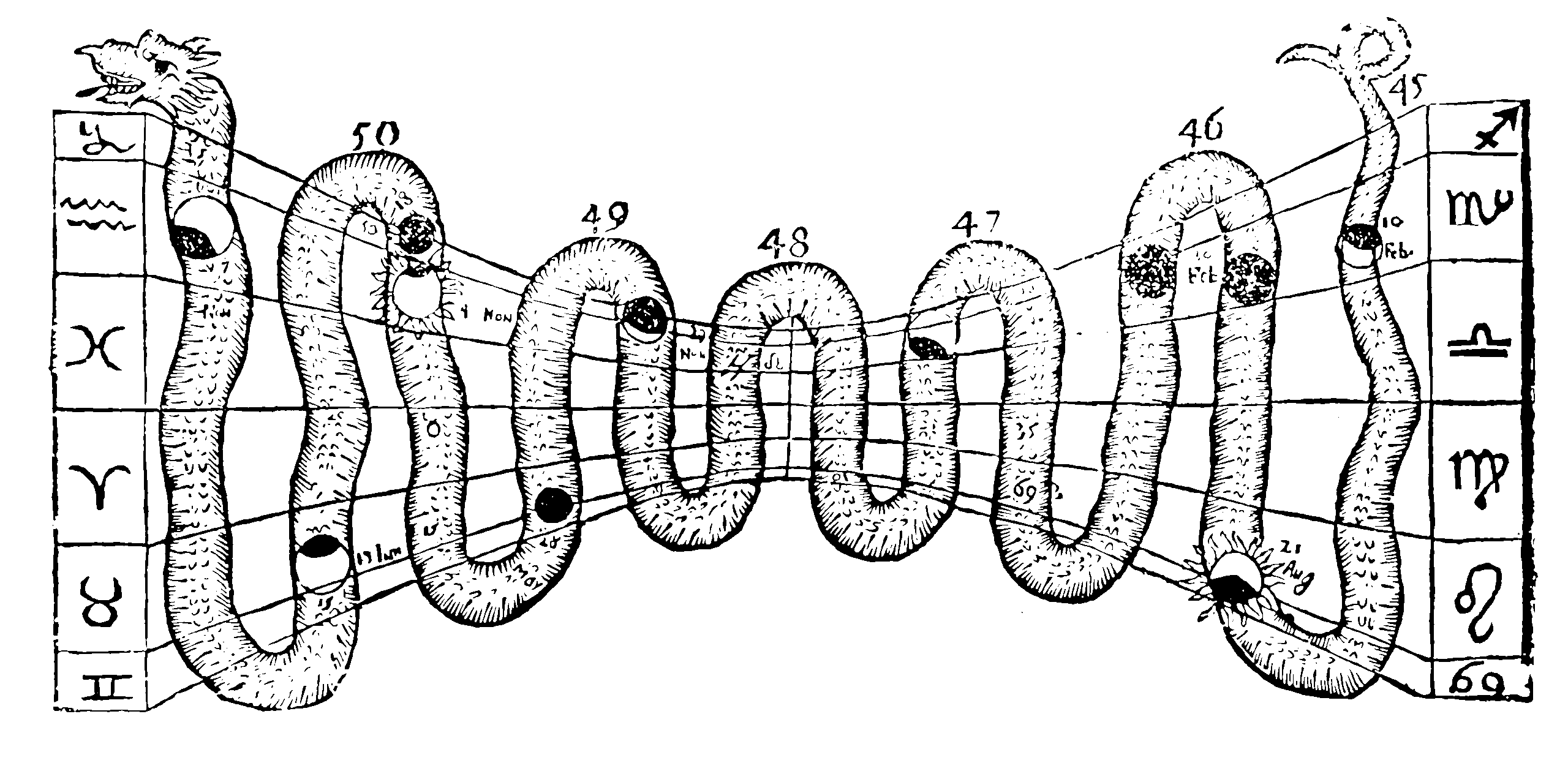# Deep sets

## invariant and equivariant functionsNeural learning of exchangeable functions, a certain type of symmetry constraint or respecting projectivity. Why might we do this? Well, for example, a learnable statistic of i.i.d observations is an exchangeable function of the data.

TBD

## Permutation invariant observations

Zaheer et al. (2017) is a short and beautiful paper.

A function $$f: 2^{\mathfrak{X}} \rightarrow \mathcal{Y}$$ acting on sets must be permutation invariant to the order of objects in the set, i.e. for any permutation $$\pi: f\left(\left\{x_1, \ldots, x_M\right\}\right)=f\left(\left\{x_{\pi(1)}, \ldots, x_{\pi(M)}\right\}\right)$$.

We use functions like this all the time; empirical statistics are of this form this form. We also use permutation equivariant functions:

$$\mathbf{f}: \mathfrak{X}^M \rightarrow \mathcal{Y}^M$$ that upon permutation of the input instances permutes the output labels, i.e. for any permutation $$\pi$$ : $\mathbf{f}\left(\left[x_{\pi(1)}, \ldots, x_{\pi(M)}\right]\right)=\left[f_{\pi(1)}(\mathbf{x}), \ldots, f_{\pi(M)}(\mathbf{x})\right]$

The main results of Zaheer et al. (2017) are:

Theorem 2: A function $$f(X)$$ operating on a set $$X$$ having elements from a countable universe, is a valid set function, i.e., invariant to the permutation of instances in $$X$$, iff it can be decomposed in the form $$\rho\left(\sum_{x \in X} \phi(x)\right)$$, for suitable transformations $$\phi$$ and $$\rho$$.

This is not proven in general for uncountable universes, but the authors assert it is true if some sane topology is applied to the space.

Equivariance:

Next, we analyze the equivariant case when $$\mathfrak{X}=\mathcal{Y}=\mathbb{R}$$ and $$\mathbf{f}$$ is restricted to be a neural network layer. The standard neural network layer is represented as $$\mathbf{f}_{\Theta}(\mathbf{x})=\boldsymbol{\sigma}(\Theta \mathbf{x})$$ where $$\Theta \in \mathbb{R}^{M \times M}$$ is the weight vector and $$\sigma: \mathbb{R} \rightarrow \mathbb{R}$$ is a nonlinearity such as sigmoid function. The following lemma states the necessary and sufficient conditions for permutation-equivariance in this type of function. Lemma 3: The function $$\mathbf{f}_{\Theta}: \mathbb{R}^M \rightarrow \mathbb{R}^M$$ defined above is permutation equivariant iff all the offdiagonal elements of $$\Theta$$ are tied together and all the diagonal elements are equal as well. That is, $$\Theta=\lambda \mathbf{I}+\gamma\left(\mathbf{1 1}^{\top}\right) \quad \lambda, \gamma \in \mathbb{R} \quad \mathbf{1}=[1, \ldots, 1]^{\top} \in \mathbb{R}^M \quad \mathbf{I} \in \mathbb{R}^{M \times M}$$ is the identity matrix This result can be easily extended to higher dimensions, i.e., $$\mathfrak{X}=\mathbb{R}^d$$ when $$\lambda, \gamma$$ can be matrices.

Original implementation is here: manzilzaheer/DeepSets; imeplentation in terms of NNs is fairly obvious; we just make $$\phi$$ and $$\rho$$ into NNs.

See also Murphy et al. (2022) and Sainsbury-Dale, Zammit-Mangion, and Huser (2022).

Great explainer by the authors of Wagstaff et al. (2019), which is an essential update to Zaheer et al. (2017). DeepSets: Modeling Permutation Invariance. Update: That same blog post without broken image links. tl;dr for continuous sets we need to know an upper bound on set size.

## Neural statistics

Related results in Edwards and Storkey (2017). Not much cross-citation between these lineages; I wonder about the overlap.

## Limited exchangeability

As seen in graph inference.

Michael Larionov:

## Attention

Some Attention networks, in particular transformers, I have been informed by my colleagues Tom Blau Xuesong Wang, are worth investigating for permutation invariance. Indeed Fabian Fuchs et al note that

Self-attention via {keys, queries, and values} as in the Attention Is All You Need paper is closely linked to Deep Sets. Self-attention is itself permutation-invariant unless you use positional encoding as often done in language applications. `In a way, self-attention “generalises” the summation operation as it performs a weighted summation of different attention vectors. You can show that when setting all keys and queries to 1.0, you effectively end up with the Deep Sets architecture.

### No comments yet. Why not leave one?

GitHub-flavored Markdown & a sane subset of HTML is supported.